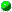`All Packages  Class Hierarchy  This Package  Previous  Next  Index`

# Class logic.BooleanTupleFactory

```java.lang.Object
|
+----logic.BooleanTupleFactory
```

public class BooleanTupleFactory
extends Object

##BooleanTupleFactory()

##computeIndicesOfStarredCols(String)
Places the positions of '*' in s into an array.countStars(String)
Counts the number of '*'s that occur in scountTuples(String)
Counts the number of boolean strings (like "TFFTF") that will be derived from s.getBoolean(char)
Converts 'T' and 'F' to the corresponding BooleansgetTuple(String)
This method returns a vector of Booleans, wrapped in a BooleanTuple, that corresponds to a boolean String like "TTFFF".getTuples(String)
This method generates the array of BooleanTuples that is generated from the boolean String tf (like "TT**F") -- a String that may contain '*'s.main(String[])
The purpose of this main method is to verify that the truthTable(..) method produces correct output.obtainBoolChar(int, int, char, int[])
If character c is just 'T' or 'F', this method just returns c.truthTable(int, int, int)
This function computes the truth value (true = 0, false = 1) that appears in a standard truth table having numStarCols propositional symbols, where starCol is the requested column index and row is the requested row index.

##BooleanTupleFactory
``` public BooleanTupleFactory()
```

##computeIndicesOfStarredCols
``` private static int[] computeIndicesOfStarredCols(String s)
```
Places the positions of '*' in s into an array. Returns an empty (non-null) array if there are no '*'s.countStars
``` private static int countStars(String s)
```
Counts the number of '*'s that occur in scountTuples
``` private static int countTuples(String s)
```
Counts the number of boolean strings (like "TFFTF") that will be derived from s. If s has no stars, the return value is 1. If 1 star, the value is 2. In general, the return value is 2^num_starsgetBoolean
``` private static Boolean getBoolean(char c)
```
Converts 'T' and 'F' to the corresponding BooleansgetTuple
``` private static BooleanTuple getTuple(String tf) throws NestingTooDeepException
```
This method returns a vector of Booleans, wrapped in a BooleanTuple, that corresponds to a boolean String like "TTFFF". Used by getTuples(..). This method does not handle Strings that contain stars, and assumes that all characters in tf are either 'T' or 'F'getTuples
``` public static BooleanTuple[] getTuples(String tf) throws NestingTooDeepException
```
This method generates the array of BooleanTuples that is generated from the boolean String tf (like "TT**F") -- a String that may contain '*'s. We first generate all possible boolean strings that don't have stars, then convert to BooleanTuples.

For example, if tf is "TT**F", this method first generates an array of four non-star boolean Strings as follows:

```     0:  "TTTTF"
1:  "TTTFF"
2:  "TTFTF"
3:  "TTFFF"
```
These four rows are thought of as a 2-d array; the 0th "column" in this example would be
```     T
T
T
T
```
In order to fill in the '*'s with all possible T's and F's, we use the truthTable(..) method. NOTE: Error handling is not being done in case conversion fails for some reason.main
``` public static void main(String args[])
```
The purpose of this main method is to verify that the truthTable(..) method produces correct output. In body of main, we attempt to output the entire truth table for 3 propositional symbols (with true = 0, false = 1). Output appears in a file c:\out.txt.obtainBoolChar
``` private static char obtainBoolChar(int col,
int row,
char c,
int indicesOfStarredCols[])
```
If character c is just 'T' or 'F', this method just returns c. If c is a star, however, then this method converts the column index of this star to the index of the array indicesOfStarredCols that contains it, and then calls truthTable(..) to determine whether, for the given column and row, the value should be 'T' or 'F' NOTE: No error handling is done in case incorrect data is being passed around.truthTable
``` public static int truthTable(int starCol,
int row,
int numStarCols)
```
This function computes the truth value (true = 0, false = 1) that appears in a standard truth table having numStarCols propositional symbols, where starCol is the requested column index and row is the requested row index. For instance, for three prop symbols, the truth table looks like this:
```      T   T   T
F   T   T
T   F   T
F   F   T
T   T   F
F   T   F
T   F   F
F   F   F
```
The way the algorithm works is this: To compute values in a truth table having n columns, the algorithm makes use of the fact that to obtain the values in columns 0...n-2, you can use computations for the case of n-1 columns. This just leaves the n-1st column to be computed. But (as you can see from the example) the n-1st column is easy to compute -- return value 0 if the requested row is in the first half of the rows (i.e., less than 2^(total_num_columns-1)), or value 1 if it's in the second half.

Parameters:
starCol - - the index of the requested column
row - - the index of the requested tow
numStarCols - - total number of columns, which is the total num of prop symbols
Returns:
int - either 0 (true) or 1 (false)

`All Packages  Class Hierarchy  This Package  Previous  Next  Index`